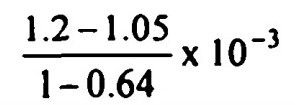top of pageSearch

# Fluid Mechanics (261-280)

Updated: Apr 22, 2020

261.discharge passing between the streamlines through the points (1,3 ) and (3,3) is

12 units

262. A model of a weir made to a horizontal scale of 1/40 and vertical scale of 1/9

discharges 1 litre/sec. Then the discharge in the prototype is estimated as 1080 lps

263. Laminar flow occurs between extensive stationary plates. The kinetic energy

correction factor is nearly 2.0

264. In steady laminar flow of a liquid through a circular pipe of internal diameter D, carrying a constant discharge, the hydraulic gradient is inversely proportional to D

265. The ratio of the coefficient of friction drag in laminar boundary layer compared to that in turbulent boundary layer is proportional to266. The overall drag coefficient of an aircraft of weight W and wing area S is given by

where a and b are constants. The maximum drag in horizontal flight will be 2W√ab

267. For laminar flow between parallel plates separated by a distance 2h, head loss

varies inversely as h³

268.A penstock is 2000 m long and the velocity of pressure wave in it is 1000

m/s. Water hammer pressure head for instantaneous closure of value at the

downstream end of pipe is 60 m. If the valve is closed in 4 sees, then the peak

water hammer pressure head is equal to 60 m

269. In an open channel of wide rectangular section with constant n value, the bed slope is 1.2 x 10⁻³ the local friction slope at a section is 1.05 x 10⁻³, the local

Froude number of the flow is 0.8. The local rate of variation of depth with

longitudinal distance along the flow direction is270. Before passage of a surge, the depth and velocity of flow at a section are 1.8 m and 3.72 m/s and., after passage, they are 0.6 m and 7.56 m/s respectively. The speed of the surge is +1.8 m/s

271. A turbine works at 20 m head and 500 rpm speed. Its 1:2 scale model to be tested at a head of 20 m should have a rotational speed of nearly 1000 rpm

272.Which one of the following statements regarding reciprocating pump is correct?-Air vessel reduces the acceleration head and consequently reduces the effect of friction head also

273.The correct sequence, in the direction of the flow of water for installations in a hydro-power plant is reservoir, pen stock, surge tank turbine

274. There are four variables, namely, E (volume modulus of elasticity), p (pres

sure per unit area), g (acceleration due to gravity) and μ (viscosity of water). They

are associated with Mach, Euler, Froude and Reynolds numbers, respectively, in

the order p, E, μ, g

275 Which one of the following pressure units represents the least pressure ?

Millibar

276.Given that, as flow takes place between two parallel static plates, the velocity

midway between the plates is 2 m/s, the Reynolds number is 1200 and the distance between the plates is 10 cm. Which of the following statements are true ? The rate of flow is 0.1 m³/s/metre width,The energy correction factor is 2.0

277. The displacement thickness of a boundary layer is the distance by which the main flow is to be shifted from the boundary to maintain the continuity equation

278 Tarbulent flow - Mixing length

Laminar flow - Hagan Poiseuille equation Lift on an aero-foil- Circulation Boundary layer- Momentum integeral equation

279. For laminar flow in a pipe carrying a given discharge, the height of surface

roughness is doubled. In such a case, Darcy-Weisbach friction factor will

remain unchanged

280. Two small orifices A and B of diameters 1 cm and 2 cm, respectively, are placed on the sides of a tank at depths of h and h below the open liquid surface. If the discharges through A and B are equal, then the ratio of h₁ and h₂ (assuming equal C𝒹 values) will be 16:1